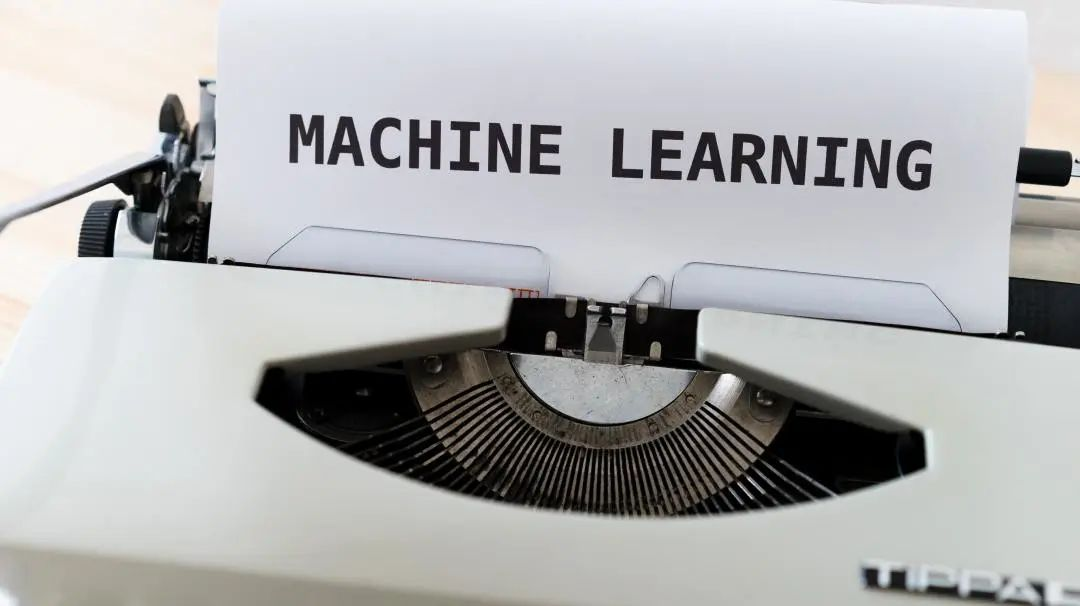# Streamlit 数据科学必备工具

Dec 09, 2021 by Zhang in  BlogStreamlit是一个开源库，致力于以更高效、更灵活的方式可视化数据，并分析结果，帮助数据科学家在短时间内开发机器学习 (ML) 可视化仪表板。只需几行代码，我们就可以构建并部署强大的数据应用程序。

• 1. Streamlit是一个免费的开源库。
• 2. 和安装其他python 包一样， Streamlit的安装非常简单。
• 3. Streamlit学起来很容易，无需要任何 Web 开发经验，只需对 Python 有基本的了解，就足以构建数据应用程序。
• 4. Streamlit与大部分机器学习框架兼容，包括 Tensorflow 和 Pytorch、Scikit-learn 和可视化库，如 Seaborn、Altair、Plotly 等。

Streamlit需要以下应用程序和包。

• 1. Python — 至少是 Python 3.7 或更高版本。
• 2. pip — 我们可以在终端或使用代码编辑器安装 pip。
• 3. Streamlit — 在启动 Streamlit 应用程序之前，我们必须安装 Streamlit 库。在终端中执行以下命令，安装streamlit。
``pip install streamlit``

• 1. 要创建基本的 streamlit 应用程序，你需要创建一个新的 Python 文件，文件名任意，例如 app.py，然后保存。
• 2. 然后使用安装的 IDE 或文本编辑器打开新创建的文件。
• 3. 在新的 python 文件中使用以下代码。
``````# Importing streamlit
import streamlit as st
# Add title and Write in
st.title('This is our First application with streamlit')
st.write("Streamlit is an awesome new tool that hepls to solve the problems quickly")``````
• 4. 在终端中使用以下命令运行应用程序。
``````# this will help to run the streamlit application
streamlit run app.py``````
• 5. 本地主机超链接将出现在终端中。在浏览器中复制该链接，或单击该链接，获得输出结果，如下所示：

``````import numpy as np
import pandas as pd
import matplotlib.pyplot as plt
import seaborn as sns
import plotly.express as px
import streamlit as st
from bokeh.plotting import figure
import altair as alt``````

``````df = pd.read_csv("Give the path of directory where you saved your csv file.")
#st.write will help to write data in application and head() will show the head of data.

``````st.title('Line_Chart,Bar_chart,Area_chart')
df_dbh_grouped=pd.DataFrame(df.groupby(['flipper_length_mm']).count()['bill_length_mm'],)
df_dbh_grouped.columns=["flipper and bill length"]
# To draw line chart
st.line_chart(df_dbh_grouped)
# To draw bar chart
st.bar_chart(df_dbh_grouped)
# To draw area chart
st.area_chart(df_dbh_grouped)``````

``````import streamlit as st
import matplotlib.pyplot as plt
import seaborn as sns

st.title("Seaborn and Matplotlib Histograms")

# Making Saeborn Chat
fig_sb, ax_sb = plt.subplots()
ax_sb = sns.histplot(df['flipper_length_mm'])
plt.xlabel('Flipper_length_mm')
st.pyplot(fig_sb)

# Making Matplotlib chart
fig_mp, ax_mpl = plt.subplots()
ax_mp = plt.hist(df['flipper_length_mm'])
plt.xlabel('Flipper_length_mm')
plt.ylabel('Count')
st.pyplot(fig_mp)``````

Plotly

Plotly 是一个开源库，提供图表类型列表，和带有回调功能的工具制作仪表板。我们将使用 Plotly 可视化数据。导入 Plotly 库，然后使用以下代码绘制直方图：

``````#importnig plotly
import plotly.express as px
st.title('Plotly Penguins')
fig=px.histogram(df["body_mass_g"])
st.plotly_chart(fig)``````

Bokeh

Bokeh 是一个 Python 数据可视化库，可生成快速交互式图表和绘图。我们使用Bokeh库创建散点图，代码如下：

``````import streamlit as st
from bokeh.plotting import figure
st.title('Bokeh Penguins')
scatterplot = figure(title = 'Bokeh Scatterplot')
scatterplot.scatter(df['bill_length_mm'], df['bill_depth_mm'])
scatterplot.yaxis.axis_label = "bill_length_mm"
scatterplot.xaxis.axis_label = "bill_depth_mm"
st.bokeh_chart(scatterplot)``````

Altair

Altair 是一个 Vega-Lite Python 接口，帮助你在 Python 中指定 Vega-Lite 图表。 通过Altair 库，以下代码将帮助你绘制条形图：

``````import altair as alt
import streamlit as st
st.title('Altair Penguins')
fig = alt.Chart(df).mark_bar().encode(x = 'species', y= 'body_mass_g').\
properties(width=600,height=400)
st.altair_chart(fig)``````

``````st.subheader('Scatter plotting with selection of category')
# Select box  is a Display interactive widget
select_species = st.selectbox('Chosse the name of any species ',[ 'Gentoo', 'Chinstrap','Adelie'])
selected_x_variable = st.selectbox('Choose  x variable for x axis',['bill_length_mm', 'bill_depth_mm', 'flipper_length_mm',
'body_mass_g'])
selected_y_var = st.selectbox('Choose y variable for y axis',['bill_length_mm', 'bill_depth_mm', 'flipper_length_mm',
'body_mass_g'])
p_df = df[df['species'] == select_species]
fig, ax = plt.subplots()
ax=plt.scatter(x = p_df[selected_x_variable],
y = p_df[selected_y_var])
plt.xlabel(selected_x_variable)
plt.ylabel(selected_y_var)
st.pyplot(fig)``````## 机器学习工程师的职业道路

Aug 09, 2023## 数据科学家，知道这些统计知识就对了

Sep 06, 2021May 11, 2023